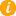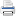# Math K-Plus

Math TestMath QuizQuiz Results• Option 2. Solve a calculator generated math problem. First press the NEW PROBLEM button. Next enter your answer, in to the calculator. Lastly, press the ADD button and then compare your answer with the calculator's.

Field Name Multiply Values
Multiplicand: You can enter up to 2 digits
Multiplier: You can enter only 1 digit
×After learning Second Grade Multiplication Calculator, you can move to the next step in multiplication math: Third Grade Multiplication Calculator.

+92
× +5
—————

92
×  5
—————

1
92
×  5
—————
0

41
92
×  5
—————
60

92
×  5
—————
460